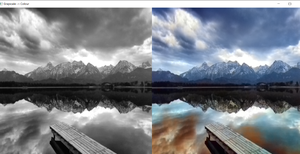# Black and white image colorization with OpenCV and Deep Learning

• Last Updated : 09 Mar, 2022

In this article, we’ll create a program to convert a black & white image i.e grayscale image to a colour image. We’re going to use the Caffe colourization model for this program. And you should be familiar with basic OpenCV functions and uses like reading an image or how to load a pre-trained model using dnn module etc. Now let’s discuss the procedure that we’ll follow to implement the program.

## Steps:

1. Load the model and the convolution/kernel points
2. Read and preprocess the image
3. Generate model predictions using the L channel from our input image
4. Use the output -> ab channel to create a resulting image

What is the L channel and ab channel? Basically like RGB colour space, there is something similar, known as Lab colour space. And this is the basis on which our program is based. Let’s discuss what it is briefly:

### What is Lab Colour Space?

Like RGB, lab colour has 3 channels L, a, and b. But here instead of pixel values, these have different significances i.e :

• L-channel: light intensity
• a channel: green-red encoding
• b channel: blue-red encoding

And In our program, we’ll use the L channel of our image as input to our model to predict ab channel values and then rejoin it with the L channel to generate our final image.

Below is the implementation of all the steps I have mentioned above.

## Python3

 `import` `numpy as np``import` `cv2``from` `cv2 ``import` `dnn` `#--------Model file paths--------#``proto_file ``=` `'Model\colorization_deploy_v2.prototxt'``model_file ``=` `'Model\colorization_release_v2.caffemodel'``hull_pts ``=` `'Model\pts_in_hull.npy'``img_path ``=` `'images/img1.jpg'``#--------------#--------------#` `#--------Reading the model params--------#``net ``=` `dnn.readNetFromCaffe(proto_file,model_file)``kernel ``=` `np.load(hull_pts)``#-----------------------------------#---------------------#` `#-----Reading and preprocessing image--------#``img ``=` `cv2.imread(img_path)``scaled ``=` `img.astype(``"float32"``) ``/` `255.0``lab_img ``=` `cv2.cvtColor(scaled, cv2.COLOR_BGR2LAB)``#-----------------------------------#---------------------#` `# add the cluster centers as 1x1 convolutions to the model``class8 ``=` `net.getLayerId(``"class8_ab"``)``conv8 ``=` `net.getLayerId(``"conv8_313_rh"``)``pts ``=` `kernel.transpose().reshape(``2``, ``313``, ``1``, ``1``)``net.getLayer(class8).blobs ``=` `[pts.astype(``"float32"``)]``net.getLayer(conv8).blobs ``=` `[np.full([``1``, ``313``], ``2.606``, dtype``=``"float32"``)]``#-----------------------------------#---------------------#` `# we'll resize the image for the network``resized ``=` `cv2.resize(lab_img, (``224``, ``224``))``# split the L channel``L ``=` `cv2.split(resized)[``0``]``# mean subtraction``L ``-``=` `50``#-----------------------------------#---------------------#` `# predicting the ab channels from the input L channel` `net.setInput(cv2.dnn.blobFromImage(L))``ab_channel ``=` `net.forward()[``0``, :, :, :].transpose((``1``, ``2``, ``0``))``# resize the predicted 'ab' volume to the same dimensions as our``# input image``ab_channel ``=` `cv2.resize(ab_channel, (img.shape[``1``], img.shape[``0``]))`  `# Take the L channel from the image``L ``=` `cv2.split(lab_img)[``0``]``# Join the L channel with predicted ab channel``colorized ``=` `np.concatenate((L[:, :, np.newaxis], ab_channel), axis``=``2``)` `# Then convert the image from Lab to BGR``colorized ``=` `cv2.cvtColor(colorized, cv2.COLOR_LAB2BGR)``colorized ``=` `np.clip(colorized, ``0``, ``1``)` `# change the image to 0-255 range and convert it from float32 to int``colorized ``=` `(``255` `*` `colorized).astype(``"uint8"``)` `# Let's resize the images and show them together``img ``=` `cv2.resize(img,(``640``,``640``))``colorized ``=` `cv2.resize(colorized,(``640``,``640``))` `result ``=` `cv2.hconcat([img,colorized])` `cv2.imshow(``"Grayscale -> Colour"``, result)` `cv2.waitKey(``0``)`

### Output:image source pexels -free stock image

## What’s next?

You can try reading the original research paper which implemented this technique – http://richzhang.github.io/colorization/ or, you can create your own model instead of using a pre-trained model.

My Personal Notes arrow_drop_up